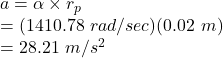## An optical disk drive in your computer can spin a disk up to 10,000 rpm (about 1045 rad/s ). If a particular disk is spun at 235.6 rad/s whi

Question

An optical disk drive in your computer can spin a disk up to 10,000 rpm (about 1045 rad/s ). If a particular disk is spun at 235.6 rad/s while it is being read, and then is allowed to come to rest over 0.167 seconds , what is the magnitude of the average angular acceleration of the disk? average angular acceleration: rad s 2 If the disk is 0.12 m in diameter, what is the magnitude of the tangential acceleration of a point 1 / 3 of the way out from the center of the disk? tangential acceleration

in progress 0
2 months 2021-07-31T09:25:45+00:00 1 Answers 0 views 0

Part(a): The angular acceleration is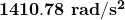.

Part(b): The linear acceleration is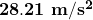.

Explanation:

Given:

The angular velocity,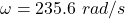Time taken,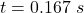Diameter of the disk,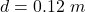Radius of the concerned point,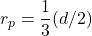.

Part(a):

The angular acceleration (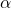) is given by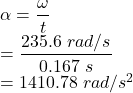Part(b):

The radius (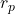) of the concerned point is given by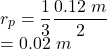Linear acceleration (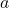) is give by